# Gesture Controlled Humanoid With Machine Learning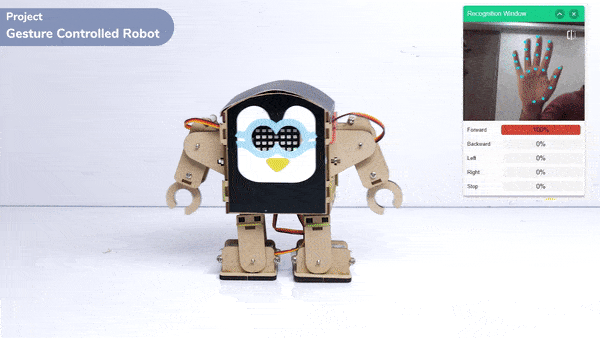Example Description
Learn to demonstrate how to use ML Environment to make a model that identifies the hand gestures and makes the humanoid move accordingly.

#### Introduction

This project demonstrates how to use Machine Learning Environment to make a machinelearning model that identifies  hand gestures and makes the humanoid move accordingly.

We are going to use the Hand Classifier of the Machine Learning Environment.  The model works by analyzing your hand position with the help of 21 data points.

#### Hand Gesture Classifier Workflow

1. Open PictoBlox and create a new file.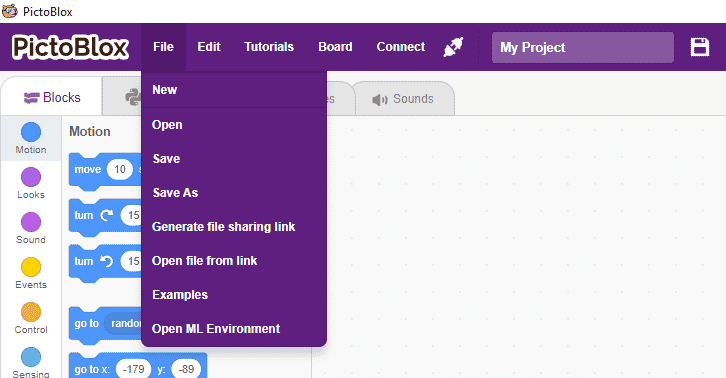2. You can click on “Machine Learning Environment” to open it.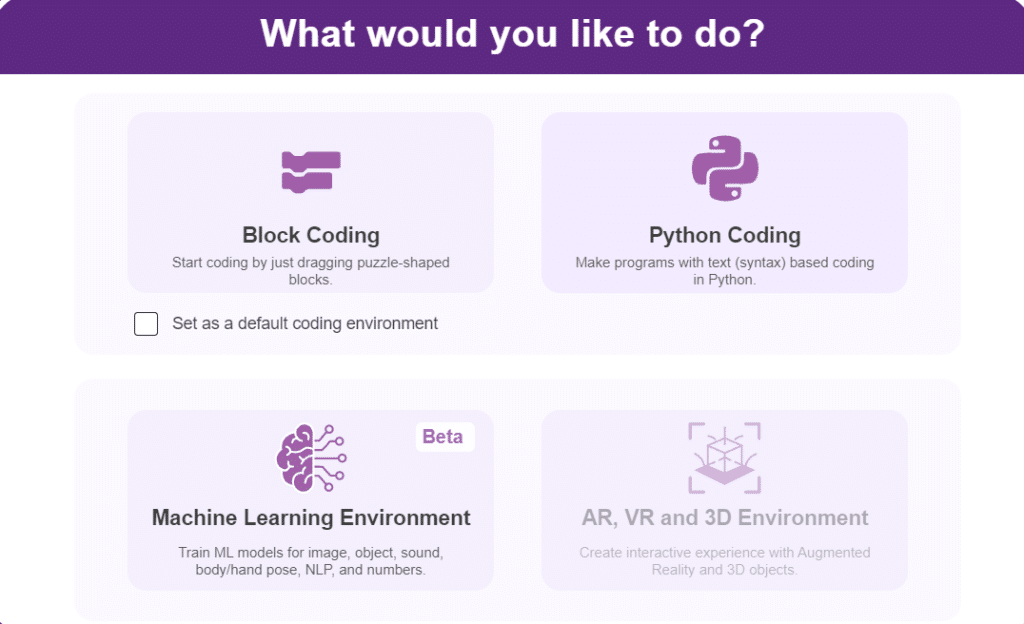3. Click on “Create New Project“.
4. A window will open. Type in a project name of your choice and select the “Hand Gesture Classifier” extension. Click the “Create Project” button to open the Hand Pose Classifier window.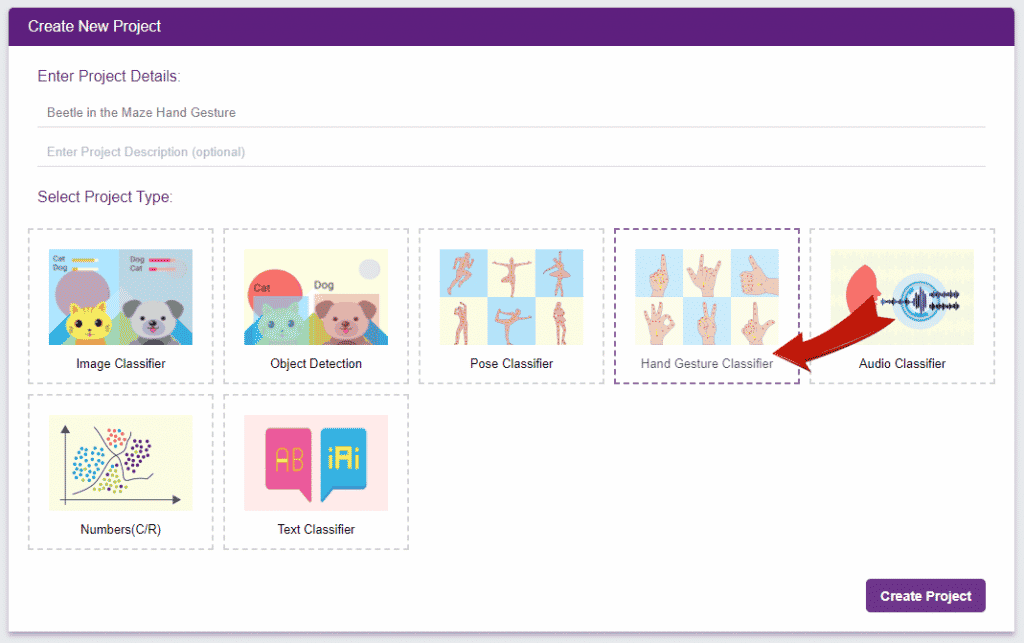5. You shall see the Classifier workflow with two classes already made for you. Your environment is all set. Now it’s time to upload the data.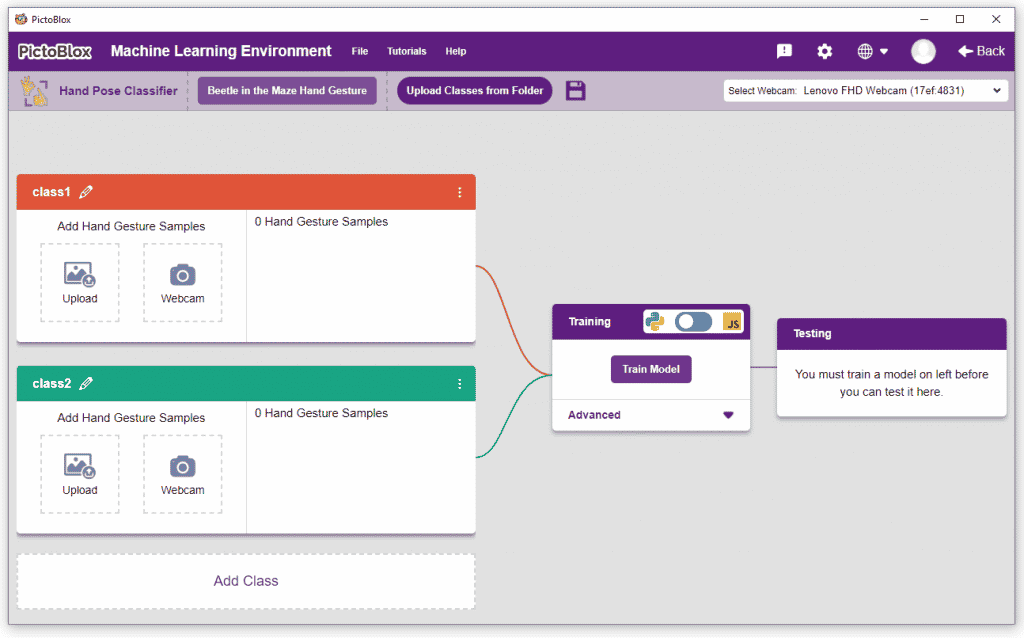##### Class in Hand Gesture Classifier

There are 2 things that you have to provide in a class:

1. Class Name: It’s the name to which the class will be referred as.
2. Hand Pose Data: This data can either be taken from the webcam or by uploading from local storage.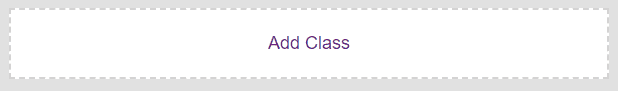Note: You can add more classes to the projects using the Add Class button.

You can perform the following operations to manipulate the data into a class.

1. Naming the Class: You can rename the class by clicking on the edit button.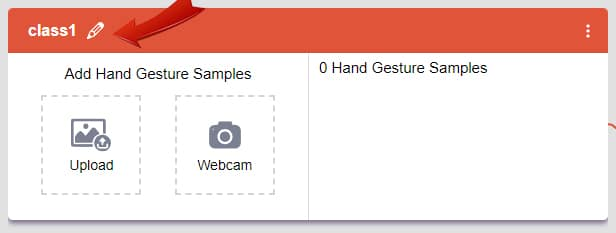2. Adding Data to the Class: You can add the data using the Webcam or by Uploading the files from the local folder.
1. Webcam: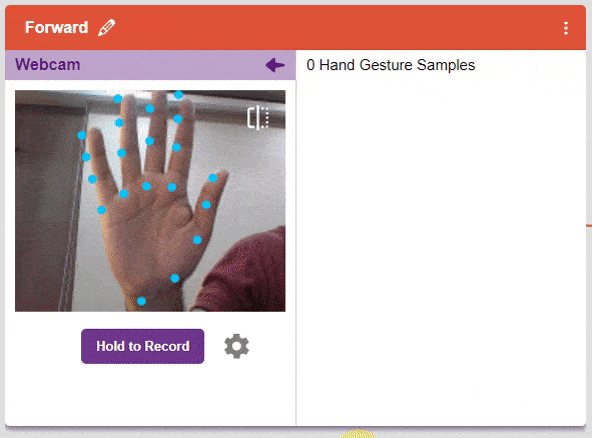Note: You must add at least 20 samples to each of your classes for your model to train. More samples will lead to better results.
##### Training the Model

After data is added, it’s fit to be used in model training. In order to do this, we have to train the model. By training the model, we extract meaningful information from the hand pose, and that in turn updates the weights. Once these weights are saved, we can use our model to make predictions on data previously unseen.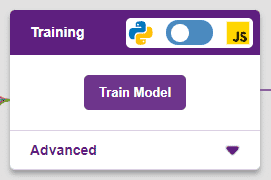The accuracy of the model should increase over time. The x-axis of the graph shows the epochs, and the y-axis represents the accuracy at the corresponding epoch. Remember, the higher the reading in the accuracy graph, the better the model. The range of the accuracy is 0 to 1.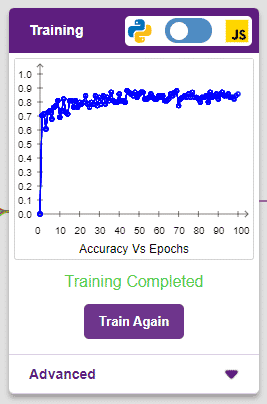##### Testing the Model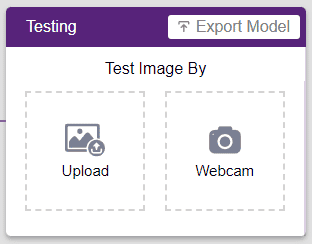To test the model, simply enter the input values in the “Testing” panel and click on the “Predict” button.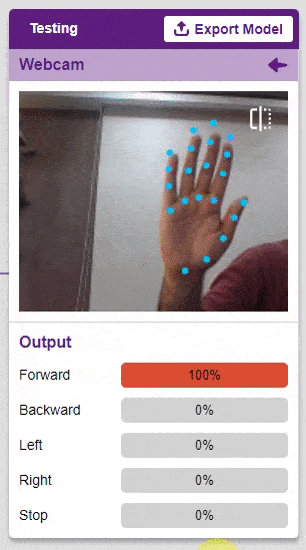The model will return the probability of the input belonging to the classes.

##### Export in Python Coding

Click on the “Export Model” button on the top right of the Testing box, and PictoBlox will load your model into the Python Coding Environment if you have opened the ML Environment in Python Coding.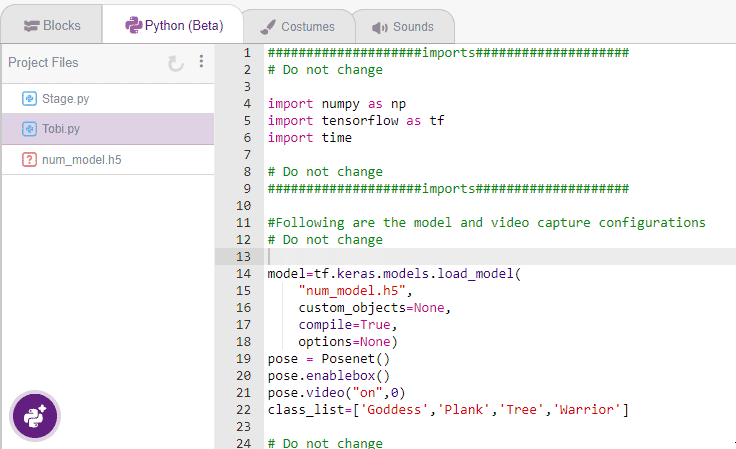#### code

The following code appears in the Python Editor of the selected sprite.

``````####################imports####################
# Do not change

import numpy as np
import tensorflow as tf

sprite=Sprite('Tobi')
import time
quarky = Quarky()
import time

humanoid = Humanoid(7, 2, 6, 3, 8, 1)

# Do not change
####################imports####################

#Following are the model and video capture configurations
# Do not change

"num_model.h5",
custom_objects=None,
compile=True,
options=None)
pose = Posenet()                                                    # Initializing Posenet
pose.enablebox()                                                    # Enabling video capture box
pose.video("on",0)                                                  # Taking video input
class_list=['forward','backward','left','right','stop']                  # List of all the classes
def runQuarky(predicted_class):
if pose.ishanddetected():
if predicted_class == "forward":
humanoid.move("forward", 1000, 1)

if predicted_class == "backward":
humanoid.move("backward", 1000, 1)

if predicted_class == "left":
humanoid.move("left", 1000, 1)

if predicted_class == "right":
humanoid.move("right", 1000, 1)

if predicted_class == "stop":
humanoid.home()
else:
quarky.stoprobot()

# Do not change
###############################################

#This is the while loop block, computations happen here
# Do not change

while True:
pose.analysehand()                                             # Using Posenet to analyse hand pose
coordinate_xy=[]

# for loop to iterate through 21 points of recognition
for i in range(21):
if(pose.gethandposition(1,i,0)!="NULL"  or pose.gethandposition(2,i,0)!="NULL"):
coordinate_xy.append(int(240+float(pose.gethandposition(1,i,0))))
coordinate_xy.append(int(180-float(pose.gethandposition(2,i,0))))
else:
coordinate_xy.append(0)
coordinate_xy.append(0)

coordinate_xy_tensor = tf.expand_dims(coordinate_xy, 0)        # Expanding the dimension of the coordinate list
predict=model.predict(coordinate_xy_tensor)                    # Making an initial prediction using the model
predict_index=np.argmax(predict, axis=0)                    # Generating index out of the prediction
predicted_class=class_list[predict_index]                      # Tallying the index with class list
print(predicted_class)
runQuarky(predicted_class)``````Note: You can edit the code to add custom code according to your requirement.

#### Logic

1. If the identified class from the analyzed image is “forward,” the humanoid will move forward at a specific speed.
2. If the identified class is “backward,” the humanoid will move backward.
3. If the identified class is “left,” the humanoid will move left.
4. If the identified class is “right,” the humanoid will move right.
5. Otherwise, the humanoid will be in the home position.
``````if pose.ishanddetected():
if predicted_class == "forward":
humanoid.move("forward", 1000, 1)

if predicted_class == "backward":
humanoid.move("backward", 1000, 1)

if predicted_class == "left":
humanoid.move("left", 1000, 1)

if predicted_class == "right":
humanoid.move("right", 1000, 1)

if predicted_class == "stop":
humanoid.home()
else:
quarky.stoprobot()``````

#### Output